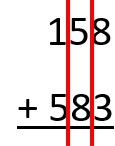# Math Worksheets Land

Math Worksheets For All Ages

# Math Worksheets Land

Math Worksheets For All Ages

# Add and Subtract Multi-Digit Whole Numbers Worksheets

This is a topic that relates a great deal to our daily lives. If there are any two math operations that we do most often, they are sums and differences. We are just starting to work at a higher scale with larger values. We will show you several different strategies to help you process these calculations much quicker. Not every technique works for everyone. We would suggest that you try them all and see which work best for you. Then stick with the strategies that work best for you. Students learn how to add and subtract mixed whole digit values in this selection of worksheets.

### Aligned Standard: Grade 4 Base Ten - 4.NBT.4

• Answer Keys - These are for all the unlocked materials above.

### Homework Sheets

I purposely off set the alignment of the first sheet to make students have to rewrite the problem. This really helps them understand the skill.

• Offset Homework 1 - Complete the following problems. The columns are off set on purpose to see what students will do to adjust.
• Homework 2 - We end up borrowing column that becomes 13-5.
• Homework 3 - Carry and borrow as needed. We would suggest the column method here.

### Practice Worksheets

You will find sum and difference on number 1. Number 2 and 3 work on each skill individually.

### Math Skill Quizzes

I mixed all the skills and number of digits used throughout each quiz.

• Quiz 1 - Get after the problems here.
• Quiz 2 - Evenly distributed problems can be found on this worksheet (quiz).
• Quiz 3 - We start out with fewer digits and advance as we go along.

### How to Add and Subtract Larger Whole Numbers

Addition and subtraction are the most basic and essential concepts in mathematics. Whether you are in grade four or in college, people have to use addition and subtraction in use in their daily lives. Addition and subtraction lay the groundwork for advanced mathematical concepts. After learning single-digit addition and subtraction, it is time that you move on to larger values that you will commonly see throughout your daily activities as you become older. The thing you have to keep in mind is that the basic principle behind these operations applies to larger and more complex values you just need to keep yourself organized while processing the calculation.

There are a number of different techniques that we can use as educators with our students to help them become comfortable with calculating sums and differences of larger values. We will examine a few here.

Strategy 1: Using Concrete Manipulatives and Place Values

The easiest way to get a grasp of the concept of addition and subtraction of multi-digit numbers is to use the concept of regrouping using concrete manipulatives. I like to use blocks that interlock in groups of ten. Such as sets of 10 Legos that are all the same color. Let’s say we were going to work up to 50, I would prepare 10 Legos in red, blue, green, orange, and yellow. This gives us 50 distinct manipulatives. It will even help them grasp the concept of carrying and borrowing. The use of color makes it very real for students.

Using this strategy, the kids can visually determine the place values. Legos get a little cumbersome here, I would replace them with ones, tens, hundreds, and thousands chips. Each chip represents one unit of that place value for example the value 825 would be represented by 8 hundreds chips, 2 tens chips, and 5 ones chips. This is a very effective stage two manipulative strategy to use.

The second most effective strategy to help students to learn to solve multi-digit addition and subtraction is to adopt the practice of splitting. Splitting is very easy to perform. All that you do is take the value and write it in expanded form. For example;

647+285

Splitting 647; 600 + 40 + 7

Splitting 285; 200 + 80 + 5

You would then add the hundreds place, tens place, and ones. This would create a final sum for you.

Strategy 3: The Column Method

This is the most traditional of strategies that you will learn in most math classrooms. It requires you to create a column for each place value. Once you create the columns, you add from the lowest place value (ones) to the highest (hundreds). Once you complete a place or go over it, you carry digits into the next column. Let’s work through this example of adding 158 and 583.We begin at the right column (ones), this gives us 11. This means that we are carrying a 1 into the tens place and dropping a 1 in the ones place. We then add the tens place which is 5 + 8 + 1 = 14. We drop the 4 in the tens place and once again carry the 1 into the hundreds place. We then add the hundreds place which is 5 + 1 + 1 = 7. This would leave us with a final answer of 741.

Unlock all the answers, worksheets, homework, tests and more!
Save Tons of Time! Make My Life Easier Now

## Thanks and Don't Forget To Tell Your Friends!

I would appreciate everyone letting me know if you find any errors. I'm getting a little older these days and my eyes are going. Please contact me, to let me know. I'll fix it ASAP.